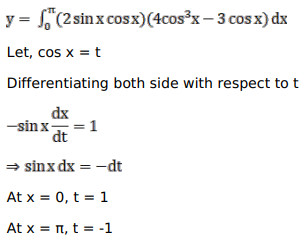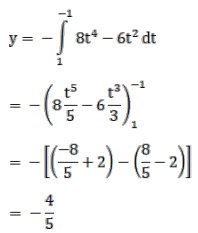# Mark against the correct answer in the following:Question:

Mark $(\sqrt{)}$ against the correct answer in the following:

$\int_{0}^{\pi}(\sin 2 x \cos 3 x) d x=?$

A. $\frac{4}{5}$

B. $-\frac{4}{5}$

C. $\frac{5}{12}$

D. $-\frac{12}{5}$

Solution: